# Work (physics)

"Mechanical work" redirects here. For other uses of "Work" in physics, see Work (electrical) and Work (thermodynamics).
Work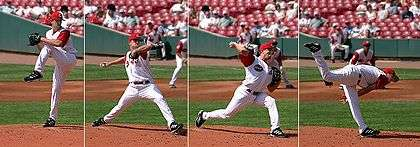A baseball pitcher does positive work on the ball by applying a force to it over the distance it moves while in his or her grip.
Common symbols
W
SI unit joule (J)
In SI base units 1 kgm2/s2
Derivations from
other quantities

W = Fs

W = τ θ

In physics, a force is said to do work if, when acting, there is a displacement of the point of application in the direction of the force. For example, when a ball is held above the ground and then dropped, the work done on the ball as it falls is equal to the weight of the ball (a force) multiplied by the distance to the ground (a displacement).

The term work was introduced in 1826 by the French mathematician Gaspard-Gustave Coriolis as "weight lifted through a height", which is based on the use of early steam engines to lift buckets of water out of flooded ore mines. The SI unit of work is the joule (J).

## Units

The SI unit of work is the joule (J), which is defined as the work expended by a force of one newton through a distance of one metre.

The dimensionally equivalent newton-metre (N⋅m) is sometimes used as the measuring unit for work, but this can be confused with the unit newton-metre, which is the measurement unit of torque. Usage of N⋅m is discouraged by the SI authority, since it can lead to confusion as to whether the quantity expressed in newton metres is a torque measurement, or a measurement of energy.

Non-SI units of work include the erg, the foot-pound, the foot-poundal, the kilowatt hour, the litre-atmosphere, and the horsepower-hour. Due to work having the same physical dimension as heat, occasionally measurement units typically reserved for heat or energy content, such as therm, BTU and Calorie, are utilized as a measuring unit.

## Work and energy

The work done by a constant force of magnitude F on a point that moves a displacement (not distance) s in the direction of the force is the product.

For example, if a force of 10 newtons (F = 10 N) acts along a point that travels 2 meters (s = 2 m), then it does the work W = (10 N)(2 m) = 20 N m = 20 J. This is approximately the work done lifting a 1 kg weight from ground level to over a person's head against the force of gravity. Notice that the work is doubled either by lifting twice the weight the same distance or by lifting the same weight twice the distance.

Work is closely related to energy. The work-energy principle states that an increase in the kinetic energy of a rigid body is caused by an equal amount of positive work done on the body by the resultant force acting on that body. Conversely, a decrease in kinetic energy is caused by an equal amount of negative work done by the resultant force.

From Newton's second law, it can be shown that work on a free (no fields), rigid (no internal degrees of freedom) body, is equal to the change in kinetic energy of the velocity and rotation of that body,The work of forces generated by a potential function is known as potential energy and the forces are said to be conservative. Therefore, work on an object that is merely displaced in a conservative force field, without change in velocity or rotation, is equal to minus the change of potential energy of the object,These formulas demonstrate that work is the energy associated with the action of a force, so work subsequently possesses the physical dimensions, and units, of energy. The work/energy principles discussed here are identical to Electric work/energy principles.

## Constraint forces

Constraint forces determine the movement of components in a system, constraining the object within a boundary (in the case of a slope plus gravity, the object is stuck to the slope, when attached to a taut string it cannot move in an outwards direction to make the string any 'tauter'). Constraint forces ensure the velocity in the direction of the constraint is zero, which means the constraint forces do not perform work on the system.

If the system doesn't change in time, they eliminate all movement in the direction of the constraint, thus constraint forces do not perform work on the system, as the velocity of that object is constrained to be 0 parallel to this force, due to this force. This only applies for a single particle system. For example, in an Atwood machine, the rope does work on each body, but keeping always the net virtual work null. There are, however, cases where this is not true.

For example, the centripetal force exerted inwards by a string on a ball in uniform circular motion sideways constrains the ball to circular motion restricting its movement away from the center of the circle. This force does zero work because it is perpendicular to the velocity of the ball.

Another example is a book on a table. If external forces are applied to the book so that it slides on the table, then the force exerted by the table constrains the book from moving downwards. The force exerted by the table supports the book and is perpendicular to its movement which means that this constraint force does not perform work.

The magnetic force on a charged particle is F = qv × B, where q is the charge, v is the velocity of the particle, and B is the magnetic field. The result of a cross product is always perpendicular to both of the original vectors, so Fv. The dot product of two perpendicular vectors is always zero, so the work W = Fv = 0, and the magnetic force does not do work. It can change the direction of motion but never change the speed.

## Mathematical calculation

For moving objects, the quantity of work/time (power) is calculated. Thus, at any instant, the rate of the work done by a force (measured in joules/second, or watts) is the scalar product of the force (a vector), and the velocity vector of the point of application. This scalar product of force and velocity is classified as instantaneous power. Just as velocities may be integrated over time to obtain a total distance, by the fundamental theorem of calculus, the total work along a path is similarly the time-integral of instantaneous power applied along the trajectory of the point of application.

Work is the result of a force on a point that moves through a distance. As the point moves, it follows a curve X, with a velocity v, at each instant. The small amount of work δW that occurs over an instant of time dt is calculated aswhere the Fv is the power over the instant dt. The sum of these small amounts of work over the trajectory of the point yields the work,where C is the trajectory from x(t1) to x(t2). This integral is computed along the trajectory of the particle, and is therefore said to be path dependent.

If the force is always directed along this line, and the magnitude of the force is F, then this integral simplifies towhere s is distance along the line. If F is constant, in addition to being directed along the line, then the integral simplifies further towhere s is the distance travelled by the point along the line.

This calculation can be generalized for a constant force that is not directed along the line, followed by the particle. In this case the dot product Fds = F cos θ ds, where θ is the angle between the force vector and the direction of movement, that isIn the notable case of a force applied to a body always at an angle of 90° from the velocity vector (as when a body moves in a circle under a central force), no work is done at all, since the cosine of 90 degrees is zero. Thus, no work can be performed by gravity on a planet with a circular orbit (this is ideal, as all orbits are slightly elliptical). Also, no work is done on a body moving circularly at a constant speed while constrained by mechanical force, such as moving at constant speed in a frictionless ideal centrifuge.

### Work done by a variable force

Calculating the work as "force times straight path segment" would only apply in the most simple of circumstances, as noted above. If force is changing, or if the body is moving along a curved path, possibly rotating and not necessarily rigid, then only the path of the application point of the force is relevant for the work done, and only the component of the force parallel to the application point velocity is doing work (positive work when in the same direction, and negative when in the opposite direction of the velocity). This component of force can be described by the scalar quantity called scalar tangential component (, whereis the angle between the force and the velocity). And then the most general definition of work can be formulated as follows:

Work of a force is the line integral of its scalar tangential component along the path of its application point.
If the force varies (e.g. compressing a spring) we need to use calculus to find the work done. If the force is given by F(x) (a function of x) then the work done by the force along the x-axis from a to b is:### Torque and rotation

A force couple results from equal and opposite forces, acting on two different points of a rigid body. The sum (resultant) of these forces may cancel, but their effect on the body is the couple or torque T. The work of the torque is calculated aswhere the Tω is the power over the instant δt. The sum of these small amounts of work over the trajectory of the rigid body yields the work,This integral is computed along the trajectory of the rigid body with an angular velocity ω that varies with time, and is therefore said to be path dependent.

If the angular velocity vector maintains a constant direction, then it takes the form,where φ is the angle of rotation about the constant unit vector S. In this case, the work of the torque becomes,where C is the trajectory from φ(t1) to φ(t2). This integral depends on the rotational trajectory φ(t), and is therefore path-dependent.

If the torque T is aligned with the angular velocity vector so that,and both the torque and angular velocity are constant, then the work takes the form,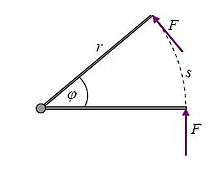A force of constant magnitude and perpendicular to the lever arm

This result can be understood more simply by considering the torque as arising from a force of constant magnitude F, being applied perpendicularly to a lever arm at a distance r, as shown in the figure. This force will act through the distance along the circular arc s = , so the work done isIntroduce the torque τ = Fr, to obtainas presented above.

Notice that only the component of torque in the direction of the angular velocity vector contributes to the work.

## Work and potential energy

The scalar product of a force F and the velocity v of its point of application defines the power input to a system at an instant of time. Integration of this power over the trajectory of the point of application, C = x(t), defines the work input to the system by the force.

### Path dependence

Therefore, the work done by a force F on an object that travels along a curve C is given by the line integral:where dx(t) defines the trajectory C and v is the velocity along this trajectory. In general this integral requires the path along which the velocity is defined, so the evaluation of work is said to be path dependent.

The time derivative of the integral for work yields the instantaneous power,### Path independence

If the work for an applied force is independent of the path, then the work done by the force is, by the gradient theorem, the potential function evaluated at the start and end of the trajectory of the point of application. Such a force is said to be conservative. This means that there is a potential function U(x), that can be evaluated at the two points x(t1) and x(t2) to obtain the work over any trajectory between these two points. It is tradition to define this function with a negative sign so that positive work is a reduction in the potential, that isThe function U(x) is called the potential energy associated with the applied force. Examples of forces that have potential energies are gravity and spring forces.

In this case, the gradient of work yieldsand the force F is said to be "derivable from a potential."

Because the potential U defines a force F at every point x in space, the set of forces is called a force field. The power applied to a body by a force field is obtained from the gradient of the work, or potential, in the direction of the velocity V of the body, that is### Work by gravity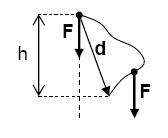Gravity F = mg does work W = mgh along any descending path

In the absence of other forces, gravity results in a constant downward acceleration of every freely moving object. Near Earth's surface the acceleration due to gravity is g = 9.8 m⋅s−2 and the gravitational force on an object of mass m is Fg = mg. It is convenient to imagine this gravitational force concentrated at the center of mass of the object.

If an object is displaced upwards or downwards a vertical distance y2y1, the work W done on the object by its weight mg is:where Fg is weight (pounds in imperial units, and newtons in SI units), and Δy is the change in height y. Notice that the work done by gravity depends only on the vertical movement of the object. The presence of friction does not affect the work done on the object by its weight.

### Work by gravity in space

The force of gravity exerted by a mass M on another mass m is given bywhere r is the position vector from M to m.

Let the mass m move at the velocity v then the work of gravity on this mass as it moves from position r(t1) to r(t2) is given byNotice that the position and velocity of the mass m are given bywhere er and et are the radial and tangential unit vectors directed relative to the vector from M to m. Use this to simplify the formula for work of gravity to,This calculation uses the fact thatThe functionis the gravitational potential function, also known as gravitational potential energy. The negative sign follows the convention that work is gained from a loss of potential energy.

### Work by a spring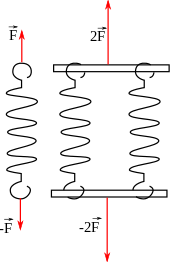Forces in springs assembled in parallel

Consider a spring that exerts a horizontal force F = (−kx, 0, 0) that is proportional to its deflection in the x direction independent of how a body moves. The work of this spring on a body moving along the space curve X(t) = (x(t), y(t), z(t)), is calculated using its velocity, v = (vx, vy, vz), to obtainFor convenience, consider contact with the spring occurs at t = 0, then the integral of the product of the distance x and the x-velocity, xvx, is (1/2)x2.

### Work by a gasWhere P is pressure, V is volume, and a and b are initial and final volumes.

## Work–energy principle

The principle of work and kinetic energy (also known as the work–energy principle) states that the work done by all forces acting on a particle (the work of the resultant force) equals the change in the kinetic energy of the particle. That is, the work W done by the resultant force on a particle equals the change in the particle's kinetic energy,,

whereandare the speeds of the particle before and after the work is done and m is its mass.

The derivation of the work–energy principle begins with Newton's second law and the resultant force on a particle which includes forces applied to the particle and constraint forces imposed on its movement. Computation of the scalar product of the forces with the velocity of the particle evaluates the instantaneous power added to the system.

Constraints define the direction of movement of the particle by ensuring there is no component of velocity in the direction of the constraint force. This also means the constraint forces do not add to the instantaneous power. The time integral of this scalar equation yields work from the instantaneous power, and kinetic energy from the scalar product of velocity and acceleration. The fact the work–energy principle eliminates the constraint forces underlies Lagrangian mechanics.

This section focuses on the work–energy principle as it applies to particle dynamics. In more general systems work can change the potential energy of a mechanical device, the heat energy in a thermal system, or the electrical energy in an electrical device. Work transfers energy from one place to another or one form to another.

### Derivation for a particle moving along a straight line

In the case the resultant force F is constant in both magnitude and direction, and parallel to the velocity of the particle, the particle is moving with constant acceleration a along a straight line. The relation between the net force and the acceleration is given by the equation F = ma (Newton's second law), and the particle displacement s can be expressed by the equationwhich follows from(see Equations of motion).

The work of the net force is calculated as the product of its magnitude and the particle displacement. Substituting the above equations, one obtains:Other derivation:Vertical displacement derivation

W = F × S = mg × h

In the general case of rectilinear motion, when the net force F is not constant in magnitude, but is constant in direction, and parallel to the velocity of the particle, the work must be integrated along the path of the particle:### General derivation of the work–energy theorem for a particle

For any net force acting on a particle moving along any curvilinear path, it can be demonstrated that its work equals the change in the kinetic energy of the particle by a simple derivation analogous to the equation above. Some authors call this result work–energy principle, but it is more widely known as the work–energy theorem:The identityrequires some algebra. From the identityand definitionit follows.

The remaining part of the above derivation is just simple calculus, same as in the preceding rectilinear case.

### Derivation for a particle in constrained movement

In particle dynamics, a formula equating work applied to a system to its change in kinetic energy is obtained as a first integral of Newton's second law of motion. It is useful to notice that the resultant force used in Newton's laws can be separated into forces that are applied to the particle and forces imposed by constraints on the movement of the particle. Remarkably, the work of a constraint force is zero, therefore only the work of the applied forces need be considered in the work–energy principle.

To see this, consider a particle P that follows the trajectory X(t) with a force F acting on it. Isolate the particle from its environment to expose constraint forces R, then Newton's Law takes the formwhere m is the mass of the particle.

#### Vector formulation

Note that n dots above a vector indicates its nth time derivative. The scalar product of each side of Newton's law with the velocity vector yieldsbecause the constraint forces are perpendicular to the particle velocity. Integrate this equation along its trajectory from the point X(t1) to the point X(t2) to obtainThe left side of this equation is the work of the applied force as it acts on the particle along the trajectory from time t1 to time t2. This can also be written asThis integral is computed along the trajectory X(t) of the particle and is therefore path dependent.

The right side of the first integral of Newton's equations can be simplified using the following identity(see product rule for derivation). Now it is integrated explicitly to obtain the change in kinetic energy,where the kinetic energy of the particle is defined by the scalar quantity,#### Tangential and normal components

It is useful to resolve the velocity and acceleration vectors into tangential and normal components along the trajectory X(t), such thatwhereThen, the scalar product of velocity with acceleration in Newton's second law takes the form.

where the kinetic energy of the particle is defined by the scalar quantity,The result is the work–energy principle for particle dynamics,This derivation can be generalized to arbitrary rigid body systems.

### Moving in a straight line (skid to a stop)

Consider the case of a vehicle moving along a straight horizontal trajectory under the action of a driving force and gravity that sum to F. The constraint forces between the vehicle and the road define R, and we haveFor convenience let the trajectory be along the X-axis, so X = (d, 0) and the velocity is V = (v, 0), then RV = 0, and FV = Fxv, where Fx is the component of F along the X-axis, soIntegration of both sides yieldsIf Fx is constant along the trajectory, then the integral of velocity is distance, soAs an example consider a car skidding to a stop, where k is the coefficient of friction and W is the weight of the car. Then the force along the trajectory is Fx = −kW. The velocity v of the car can be determined from the length s of the skid using the work–energy principle,Notice that this formula uses the fact that the mass of the vehicle is m = W/g.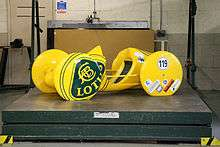Lotus type 119B gravity racer at Lotus 60th celebration.Gravity racing championship in Campos Novos, Santa Catarina, Brazil, 8 September 2010.

### Coasting down a mountain road (gravity racing)

Consider the case of a vehicle that starts at rest and coasts down a mountain road, the work-energy principle helps compute the minimum distance that the vehicle travels to reach a velocity V, of say 60 mph (88 fps). Rolling resistance and air drag will slow the vehicle down so the actual distance will be greater than if these forces are neglected.

Let the trajectory of the vehicle following the road be X(t) which is a curve in three-dimensional space. The force acting on the vehicle that pushes it down the road is the constant force of gravity F = (0, 0, W), while the force of the road on the vehicle is the constraint force R. Newton's second law yields,The scalar product of this equation with the velocity, V = (vx, vy, vz), yieldswhere V is the magnitude of V. The constraint forces between the vehicle and the road cancel from this equation because RV = 0, which means they do no work. Integrate both sides to obtainThe weight force W is constant along the trajectory and the integral of the vertical velocity is the vertical distance, therefore,Recall that V(t1)=0. Notice that this result does not depend on the shape of the road followed by the vehicle.

In order to determine the distance along the road assume the downgrade is 6%, which is a steep road. This means the altitude decreases 6 feet for every 100 feet traveled—for angles this small the sin and tan functions are approximately equal. Therefore, the distance s in feet down a 6% grade to reach the velocity V is at leastThis formula uses the fact that the weight of the vehicle is W = mg.

## Work of forces acting on a rigid body

The work of forces acting at various points on a single rigid body can be calculated from the work of a resultant force and torque. To see this, let the forces F1, F2 ... Fn act on the points X1, X2 ... Xn in a rigid body.

The trajectories of Xi, i = 1, ..., n are defined by the movement of the rigid body. This movement is given by the set of rotations [A(t)] and the trajectory d(t) of a reference point in the body. Let the coordinates xi i = 1, ..., n define these points in the moving rigid body's reference frame M, so that the trajectories traced in the fixed frame F are given byThe velocity of the points Xi along their trajectories arewhere ω is the angular velocity vector obtained from the skew symmetric matrixknown as the angular velocity matrix.

The small amount of work by the forces over the small displacements δri can be determined by approximating the displacement by δr = vδt soorThis formula can be rewritten to obtainwhere F and T are the resultant force and torque applied at the reference point d of the moving frame M in the rigid body.

1. Jammer, Max (1957). Concepts of Force. Dover Publications, Inc. p. 167; footnote 14. ISBN 0-486-40689-X.
2. Coriolis, Gustave. (1829). Calculation of the Effect of Machines, or Considerations on the Use of Engines and their Evaluation (Du Calcul de l'effet des Machines, ou Considérations sur l'emploi des Moteurs et sur Leur Evaluation). Paris: Carilian-Goeury, Libraire.
3. http://www.bipm.org/en/si/si_brochure/chapter2/2-2/2-2-2.html
4. Goldstein, Classical Mechanics, third edition. P.19
5. Resnick, Robert and Halliday, David (1966), Physics, Section 1–3 (Vol I and II, Combined edition), Wiley International Edition, Library of Congress Catalog Card No. 66-11527
6. Hugh D. Young & Roger A. Freedman (2008). University Physics (12th ed.). Addison-Wesley. p. 329. ISBN 978-0-321-50130-1.
7. J. R. Taylor, Classical Mechanics, University Science Books, 2005.
8. Andrew Pytel; Jaan Kiusalaas (2010). Engineering Mechanics: Dynamics – SI Version, Volume 2 (3rd ed.). Cengage Learning,. p. 654. ISBN 9780495295631.
9. B. Paul, Kinematics and Dynamics of Planar Machinery, Prentice-Hall, 1979.
10. E. T. Whittaker, A treatise on the analytical dynamics of particles and rigid bodies, Cambridge University Press, 1904
11. Work–energy principle

## Bibliography

• Serway, Raymond A.; Jewett, John W. (2004). Physics for Scientists and Engineers (6th ed.). Brooks/Cole. ISBN 0-534-40842-7.
• Tipler, Paul (1991). Physics for Scientists and Engineers: Mechanics (3rd ed., extended version ed.). W. H. Freeman. ISBN 0-87901-432-6.